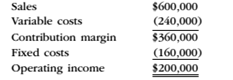Chapter 11, Problem 5SEQ

Chapter
Section
Textbook Problem

Based on the following operating data, what is the operating leverage?A. 0.8 B. 1.2 C. 1.8 D. 4.0

To determine

Concept Introduction:

Degree of operating leverage:

The Degree of operating leverage shows the relation between change in net operating income and change in sales. The formulas for degree of operating leverage are as follows:

Degree of operating leverage =  Contribution MarginOperating income

Change in operating income = Changes in sales ×Degree of operating leverage

To Calculate:

The Degree of operating leverage

Explanation

The Degree of operating leverage is calculated as follows:

 Total Contribution Margin (C) \$ 360,000

Still sussing out bartleby?

Check out a sample textbook solution.

See a sample solution

The Solution to Your Study Problems

Bartleby provides explanations to thousands of textbook problems written by our experts, many with advanced degrees!

Get Started

Find more solutions based on key concepts